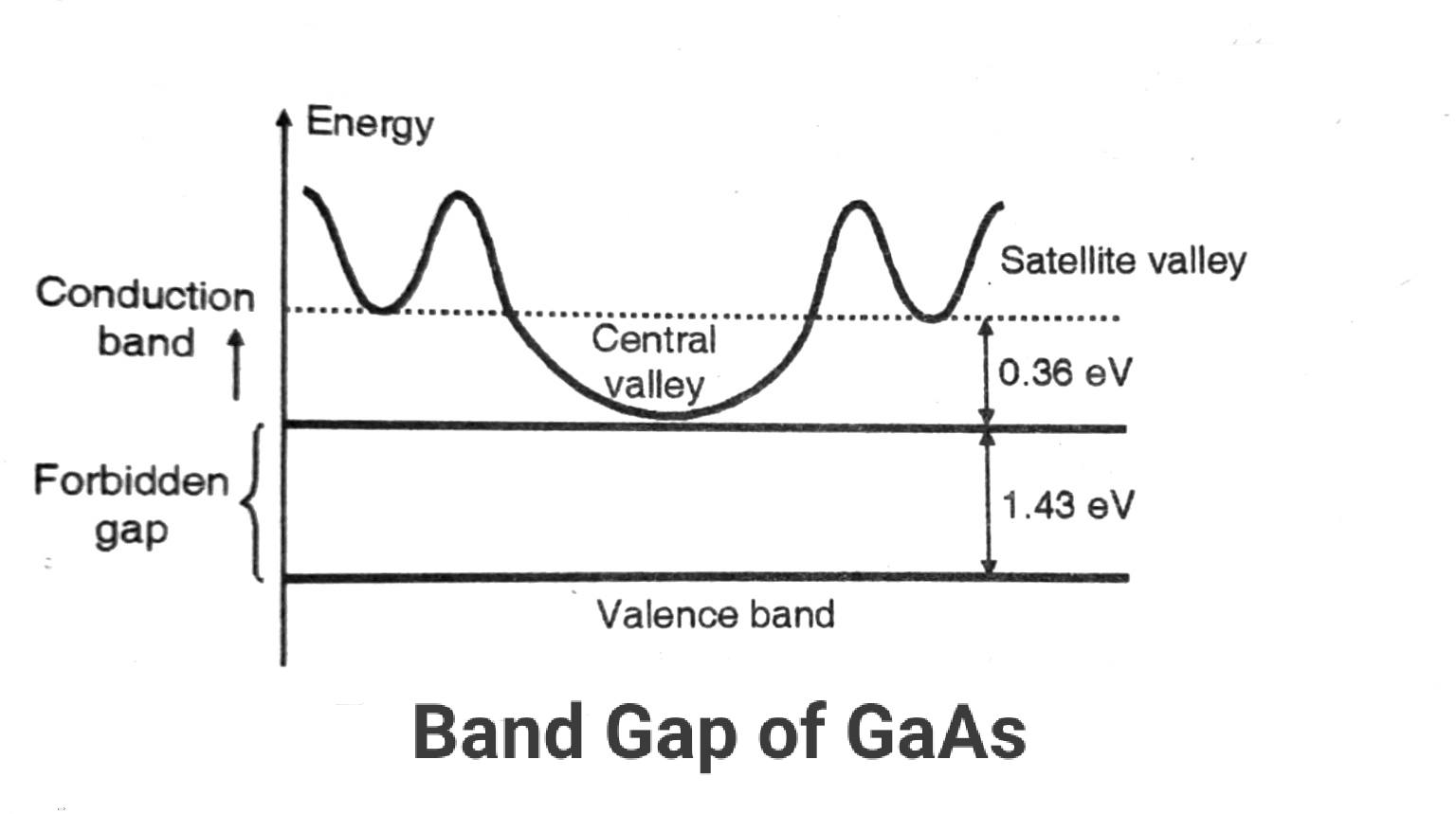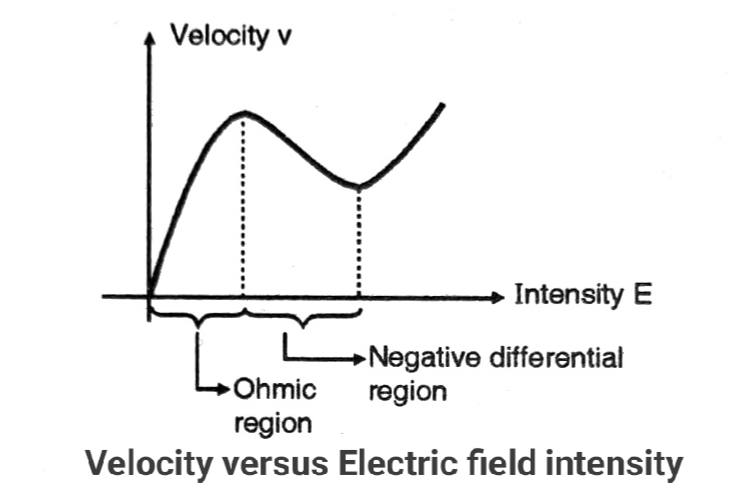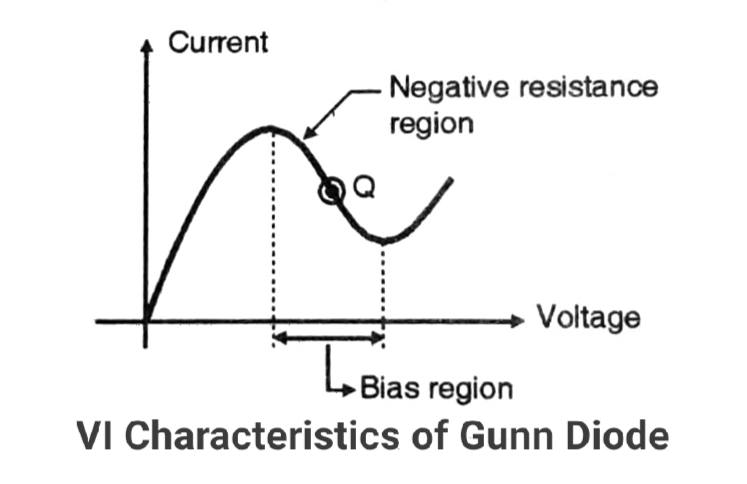# Gunn diode working principle VI characteristics & applications

Gunn diode operates on the Gunn effect which is named after its inventor J.B. Gunn. He discovered the periodic variation of current in a GaAs (gallium arsenide) rod in 1963. The microwave device that operates on the principle of transfer of electrons is called as Gunn diode.

This is one of the diodes used as a microwave detector. It uses the avalanche and transit time properties of semiconductors.

The mechanism of transferred electrons is related to the conduction band electrons. In the case of certain semiconductor devices, the conduction band electrons get shifted from a state of higher mobility to lower mobility, under the influence of a strong electric field.

## Principle of operation of Gunn Diode:

Consider the energy diagram of GaAs semiconductor, shown in Figure.

The conduction band of GaAs is divided into two levels namely the central valley and satellite valley.### Central Valley:

The central valley is 1.43 eV above the valence band. Its height is 0.36 eV. The central valley generally consists of the conduction electrons.

### Satellite valley:

The satellite valley is generally at a higher energy level than the central valley.

In each energy band the electrons have the following properties :

1. Density 2. Effective mass 3. Mobility

The effective mass and mobility of electrons are related to the valleys as follows:

Upper valley -> Higher effective mass, lower mobility

Lower valley -> Small effective mass, higher mobility

Keeping all this in mind now we shall see the mechanism of electron transfer.

### Mechanism of electron transfer:

We know that relation between mobility, drift velocity and the electric field is as follows:

`V = uE`

The current density J is given by.

`J = n.q.v = n.q.u.E`

At room temperature when the applied electric field intensity is low (E is small), then almost all the electrons are present in the lower valley i.e. at the bottom of the conduction band.

Now if the electric field intensity is increased then more photons are generated.

They will strike the electrons present at the bottom of the conduction band. This collision will transfer energy from photons to electrons.

So the kinetic energy of these electrons will increase and they start moving in the upward direction. This is called electron transfer.

If the electric field intensity is increased further the electrons move to the satellite valley.

The effective mass of electrons will increase as they move towards the satellite valley but the mobility reduces.

With further increase in electric field intensity, the energy of electrons will increase but velocity decreases. Therefore the current density J decreases.

So in the range of increasing electric field intensity, we get a negative differential coefficient (-dJ / dE).

Let u1 be the mobility of electrons in the lower valley and u2 be the mobility in the upper valley. Then the mean mobility is given by.

`u = n1u1 + n2u2 /(n1 + n2)`

And the current density is given by

`J = (n1 + n2)uqE`

So the conductivity of the GaAs rod is given by

`¶ = q( u1n1 + u2n2)`

### Velocity Vs Electric field curve:The characteristics are divided into two regions:

1. Ohmic region 2. Negative differential region

#### Ohmic region:

In this region, the electrons are present in the central valley. With an increase in the applied electric field, the velocity of electrons increases.

#### Negative differential resistance region :

In this region, the velocity decreases with an increase in electric field intensity. This is called a negative differential resistance region.

#### Modes of operation:

The modes of operation of a Gunn diode are as follows:

• The Gunn mode.
• Stable amplifier mode.
• Limited space charge accumulation mode (LSA).

Depending on the application, the Gunn diode is biased in one of the modes stated above.

The V-I characteristics of a Gunn diode are shown in Figure.The diode is biased to operate in the negative resistance region if it is to be operated as an oscillator. The bias voltage is adjusted in such a way that the diode is biased at the center of the negative resistance region.

### Applications of Gunn Diode:

Gunn diodes are generally used in power oscillators in microwave instruments.

It can be used as a pump source.

This site uses Akismet to reduce spam. Learn how your comment data is processed.